# ACT Math Practice Test 6

Difficulty Level – 3: Medium / Difficult

Directions: Solve each problem and then click on the correct answer. You are permitted to use a calculator on this test.

Congratulations - you have completed . You scored %%SCORE%% out of %%TOTAL%%. Your performance has been rated as %%RATING%%
 Question 1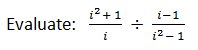A −2 B −1 C 0 D 1 E 2
Question 1 Explanation:
The correct answer is (C). This initially looks very complicated; however, recall that i is equal to the square root of −1, so i2 is equal to −1. Evaluating the numerator of the expression on the left gives us 0 and because we are dividing the first expression by the second and 0 divided by anything is 0, the entire expression evaluates to 0.
 Question 2
What is the sum of the x and y coordinates of the midpoint between the points (−2, 9) and (10, −4)?

 A 3.5 B 5 C 6.5 D 7.5 E 8
Question 2 Explanation:
The correct answer is (C). Recall midpoint formula is the average of the x values and the average of the y values in (x,y) coordinate form.

First find the x coordinate midpoint:
(10 + (−2)) ÷ 2
= 4

Then find the y coordinate midpoint:
(9 + (−4)) ÷ 2
=2.5

Add them together to get the sum:
4 + 2.5 = 6.5
 Question 3
A group of 6 students are going to have a vote to determine who should be President, Vice President, Secretary, and Treasurer with only 1 person per job. How many different groups can be formed from the 6 students?

 A 30 B 45 C 90 D 180 E 360
Question 3 Explanation:
The correct answer is (E). In approaching this problem, consider the number of options the students have for each role. As a role is taken up, there is 1 less student to fill the next role. For President there are 6 options, for Vice President 5 options, for Secretary 4 options, and for Treasurer 3 options. Multiply each of these to find 360 different groups:
6 * 5 * 4 * 3 = 360
 Question 4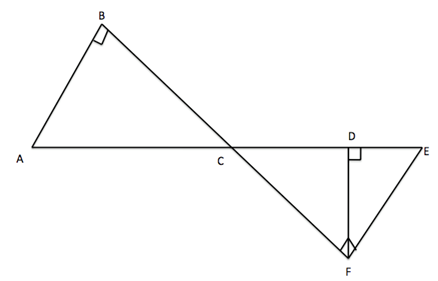In the figure above, A, C, D, and E are collinear; B, C, and F are collinear; and the angles at B, D, and F are right angles, as marked. Which of the following statements is NOT justifiable from the given information?

 A AB is parallel to EF. B BC is congruent to CF. C EF is perpendicular to BF. D Angle BCA is congruent to angle DCF. E Triangle BAC is similar to triangle DCF.
Question 4 Explanation:
The correct answer is (B). Solve this by process of elimination.

A: True: AB and EF are both perpendicular to line BCF.
C: True: Angle F is a right angle, so the two lines are perpendicular.
D: True: Angle BCA and angle DCF are vertical angles, so they must be equal.
E: True: Angle ABC = angle CDF (they are both right angles).

Angle BCA = angle DCF (see statement D). Therefore, angle BAC must equal angle DFC (since the other two angles are equal, and the three angles total to 180). Since the two triangles have three corresponding angles, they are similar. B can be false.

Although we know that triangles ABC and CDF are similar, we do not know that they are congruent. So these sides may be unequal.
 Question 5
If (3.1358)(10x) = 0.0000031358 what is the value of x?

 A −7 B −6 C −5 D 6 E 7
Question 5 Explanation:
The correct answer is (B). The easiest way to solve this problem is to count how many places the decimal moves from the number on the left to the number on the right. Our decimal moves 6 places to the left, so x = −6.
 Question 6
A triangle is composed of angles represented as:

3x + 10, −2x + 40, and x + 40

What is the value of x?

 A 45 B 50 C 55 D 60 E 65
Question 6 Explanation:
The correct answer is (A). Recall that the angles of a triangle sum to 180 degrees:
3x + 10 + −2x + 40 + x + 40 = 180
2x + 90 = 180
2x = 90
x = 45
 Question 7
Choose an expression that is equivalent to the following: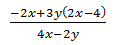A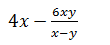B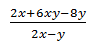C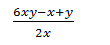DE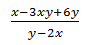Question 7 Explanation:
The correct answer is (E). Begin by distributing the 3y through the parentheses to get −2x + 6xy − 12y as the numerator. Factor out a −2 to get −2(x − 3xy+ 6y), also factor out a −2 from the denominator to get −2(−2x + y), eliminate the −2 to get numerator of x − 3xy + 6y and denominator of y − 2x.
 Question 8The curve y = 0.01x2 - 3x + 150 for 0 ≤ x ≤ 100 and the line segment from F(0,100) to G(100,0) are shown in the standard (x, y) coordinate plane provided. To the nearest integer, what is the y-coordinate for the point on the curve with x-coordinate 50?

 A 0 B 25 C 50 D 75 E 100
Question 8 Explanation:
The correct answer is (B). Plug the given x value into the given equation:
y = 0.01(502) − 3(50) + 150
y = .01(2500) − 150 + 150
y = 25 − 150 + 150
y = 25
 Question 9
If the number 89.8756 is rounded to the nearest hundredth, what will be the sum of the tenths and hundredths place of the resulting number?

 A 10 B 12 C 14 D 16 E 18
Question 9 Explanation:
The correct answer is (D). Begin by rounding the number to the nearest hundredth: 89.88. Now add the tenths place, 8, and the hundredths, 8, to get 16.
 Question 10
On the Cartesian plane, what is the distance between the points (0,0) and (5,−5)?

 A 5 B$\sqrt{50}$ C$\sqrt{60}$ D$\sqrt{75}$ E 10
Question 10 Explanation:
The correct answer is (B). One way to do this problem is to use the distance formula right away. Another way to do it is to graph the points and see that this is a right triangle with side lengths 5 and 5 so our hypotenuse (or the distance between the points) is$5\sqrt{2}$ which is equal to the square root of 50.
Once you are finished, click the button below. Any items you have not completed will be marked incorrect.
There are 10 questions to complete.
 ← List →